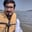Related Tags

python
pandas
columns
communitycreator

# How to get positions where values of two columns match in pandasArslan Bajwa

In Python, the pandas library includes built-in functionalities that allow you to perform different tasks with only a few lines of code. One of these functionalities is how to find positions of two matching columns.

## Input

 City1 City2 Lahore Lahore Karachi Islamabad Multan Karachi Multan Multan Lahore Islamabad

• [ 0, 3 ]

## Method

To find the positions of two matching columns, we first initialize a pandas dataframe with two columns of city names. Then we use where() of numpy to compare the values of two columns.

This returns an array that represents the indices where the two columns have the same value.

#importing pandas and numpy libraries
import pandas as pd
import numpy as np

#initializing pandas dataframe
df = pd.DataFrame({'City1': np.random.choice(['Lahore', 'Multan', 'Karachi'], 10),

#displaying initialized dataframe
print(df)

#displaying columns where values match in a dataframe
print(np.where(df.City1 == df.City2))

RELATED TAGS

python
pandas
columns
communitycreator

CONTRIBUTORArslan Bajwa
RELATED COURSES

View all Courses

Keep Exploring

Learn in-demand tech skills in half the time